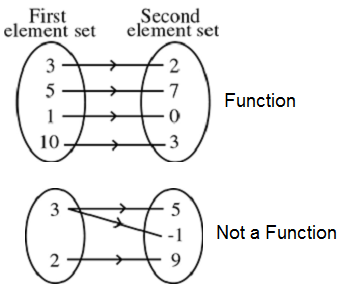# Functions

A function is a special type of relation. In a function, no two ordered pairs can have the same first element and a different second element. That is, for a function, corresponding to each first element of the ordered pairs, there must be a different second element.

Consider the set of ordered pairs (relation): {(3, 2),(5, 7),(1, 0),(10, 3)}. Here no two ordered pairs have the same first element and different second element. This relation is a function.

Consider another set of ordered pairs (relation): {(3, 5),(3, -1),(2, 9)}. Here the ordered pairs (3, 5) and (3, -1) have the same first element but different second element. This relation is not a function.### Definition of Function

A function f from a set A to B is a rule (relation) that assigns a unique element f(x) in B to each element x in A.

f: A → B

x → f(x)

To denote functions, use the letters f, g, h, etc. Thus for a function, each element of A is associated with exactly one element in B. The set A is called the domain of the function f and B is called co-domain of f. If x is in A, the element of B associated with x is called the image of x under f. The set of all images of the elements of A is called the range of the function f. The range is a subset of the co-domain.